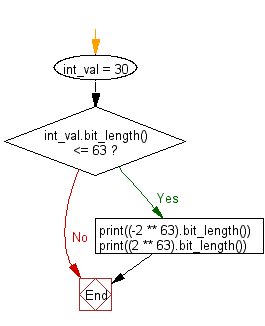﻿ Python: Check whether an integer fits in 64 bits - w3resource# Python: Check whether an integer fits in 64 bits

## Python Basic: Exercise-127 with Solution

Write a Python program to check whether an integer fits in 64 bits.

Sample Solution:-

Python Code:

``````int_val = 30
if int_val.bit_length() <= 63:
print((-2 ** 63).bit_length())
print((2 ** 63).bit_length())
```
```

Sample Output:

```64
64
```

Flowchart:## Visualize Python code execution:

The following tool visualize what the computer is doing step-by-step as it executes the said program:

Python Code Editor:

Have another way to solve this solution? Contribute your code (and comments) through Disqus.

What is the difficulty level of this exercise?

Test your Python skills with w3resource's quiz

﻿

## Python: Tips of the Day

Try-catch-else construct:

```try:
foo()
except Exception:
print("Exception occured")
else:
print("Exception didnt occur")
finally:
print("Always gets here")
```